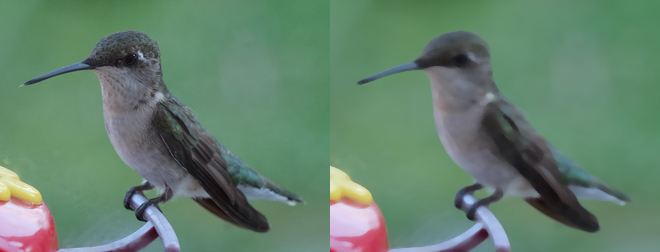# Transformation¶

Note

The image transform.jpg used in this docs is taken by Megan Trace, and licensed under CC BY-NC 2.0. It can be found the original photography from Flickr.

## Charcoal¶

New in version 0.5.3.

One of the artistic simulations, charcoal() can emulate a drawing on paper.

from wand.image import Image

with Image(filename="hummingbird.jpg") as left:
with left.clone() as right:
right.charcoal(radius=1.5, sigma=0.5)
left.extent(width=left.width*2)
left.composite(right, top=0, left=right.width)
left.save(filename="hummingbird-charcoal.jpg")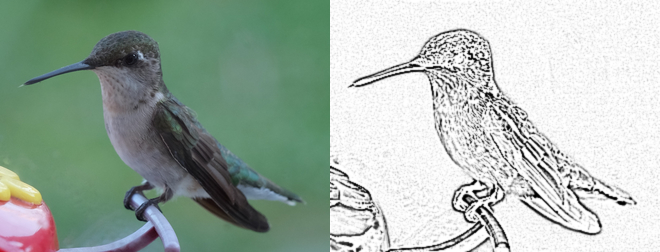## Despeckle¶

New in version 0.5.0.

Despeckling is one of the many techniques you can use to reduce noise on a given image. Also see Enhance.

from wand.image import Image

with Image(filename="hummingbird.jpg") as left:
with left.clone() as right:
right.despeckle()
left.extent(width=left.width*2)
left.composite(right, top=0, left=right.width)
left.save(filename="hummingbird-despeckle.jpg")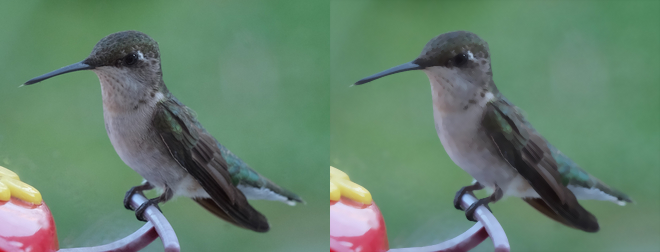## Edge¶

New in version 0.5.0.

Detects edges on black and white images with a simple convolution filter. If used with a color image, the transformation will be applied to each color-channel.

from wand.image import Image

with Image(filename="hummingbird.jpg") as left:
with left.clone() as right:
right.transform_colorspace("gray")
right.edge(1)
left.extent(width=left.width*2)
left.composite(right, top=0, left=right.width)
left.save(filename="hummingbird-edge.jpg")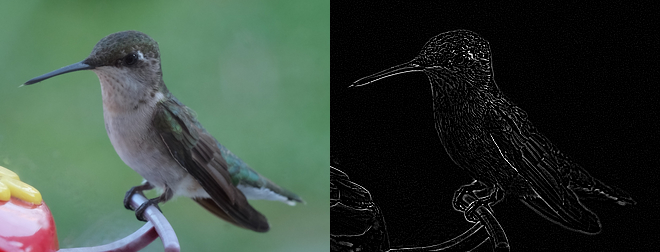## Emboss¶

New in version 0.5.0.

Generates a 3D effect that can be described as print reliefs. Like Edge, best results can be generated with grayscale image. Also see Shade.

from wand.image import Image

with Image(filename="hummingbird.jpg") as left:
with left.clone() as right:
right.transform_colorspace("gray")
right.emboss(radius=3.0, sigma=1.75)
left.extent(width=left.width*2)
left.composite(right, top=0, left=right.width)
left.save(filename="hummingbird-emboss.jpg")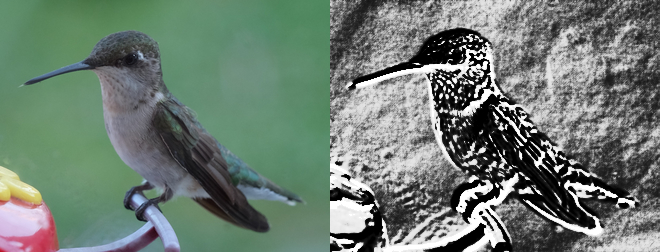## Enhance¶

New in version 0.5.0.

Reduce the noise of an image by applying an auto-filter. Also see Despeckle.

from wand.image import Image

with Image(filename="hummingbird.jpg") as left:
with left.clone() as right:
right.enhance()
left.extent(width=left.width*2)
left.composite(right, top=0, left=right.width)
left.save(filename="hummingbird-enhance.jpg")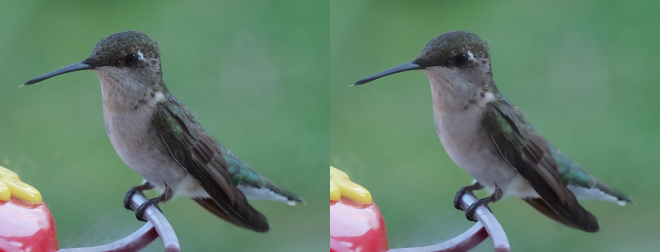## Flip and flop¶

New in version 0.3.0.

You can make a mirror image by reflecting the pixels around the central x- or y-axis. For example, where the given image transform.jpg: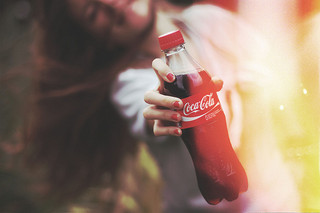The following code flips the image using Image.flip() method:

from wand.image import Image

with Image(filename='transform.jpg') as image:
with image.clone() as flipped:
flipped.flip()
flipped.save(filename='transform-flipped.jpg')


The image transform-flipped.jpg generated by the above code looks like: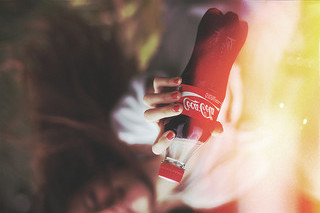As like flip(), flop() does the same thing except it doesn’t make a vertical mirror image but horizontal:

from wand.image import Image

with Image(filename='transform.jpg') as image:
with image.clone() as flopped:
flopped.flop()
flopped.save(filename='transform-flopped.jpg')


The image transform-flopped.jpg generated by the above code looks like: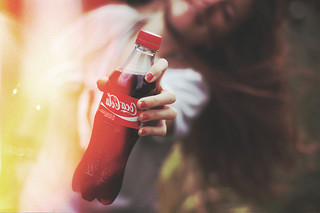## Noise¶

New in version 0.5.3.

You can add random noise to an image. This operation can be useful when applied before a blur operation to defuse an image. The types of noise can be any of the following.

• 'gaussian'
• 'impulse'
• 'laplacian'
• 'multiplicative_gaussian'
• 'poisson'
• 'random'
• 'uniform'

The amount of noise can be adjusted by passing an attenuate kwarg where the value can be between 0.0 and 1.0.

from wand.image import Image

with Image(filename="hummingbird.jpg") as left:
with left.clone() as right:
right.noise("laplacian", attenuate=1.0)
left.extent(width=left.width*2)
left.composite(right, top=0, left=right.width)
left.save(filename="hummingbird-noise.jpg")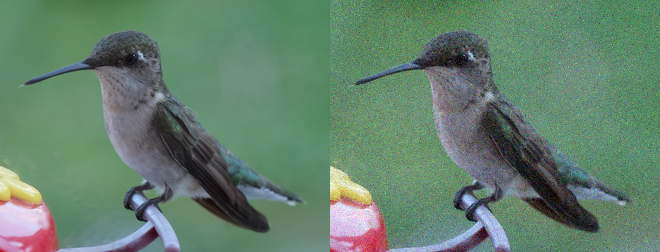## Remap¶

New in version 0.5.3.

Remap replaces all pixels with the closest matching pixel found in the affinity reference image.

from wand.image import Image

with Image(filename="hummingbird.jpg") as left:
with left.clone() as right:
with Image(width=100, height=1, pseudo="plasma:") as affinity:
right.remap(affinity)
left.extent(width=left.width*2)
left.composite(right, top=0, left=right.width)
left.save(filename="hummingbird-remap.jpg")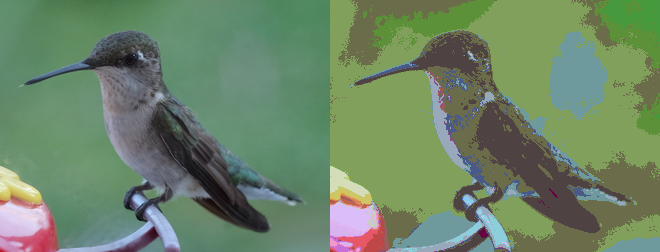## Rotation¶

New in version 0.1.8.

Image object provides a simple method to rotate images: rotate(). It takes a degree which can be 0 to 359. (Actually you can pass 360, 361, or more but it will be the same to 0, 1, or more respectively.)

For example, where the given image transform.jpg:The below code makes the image rotated 90° to right:

from wand.image import Image

with Image(filename='transform.jpg') as image:
with image.clone() as rotated:
rotated.rotate(90)
rotated.save(filename='transform-rotated-90.jpg')


The generated image transform-rotated-90.jpg looks like: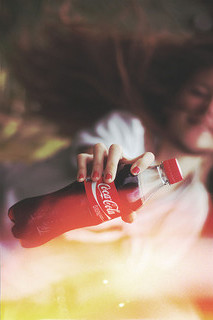If degree is not multiples of 90, the optional parameter background will help (its default is transparent):

from wand.color import Color
from wand.image import Image

with Image(filename='transform.jpg') as image:
with image.clone() as rotated:
rotated.rotate(135, background=Color('rgb(229,221,112)'))
rotated.save(filename='transform-rotated-135.jpg')


The generated image transform-rotated-135.jpg looks like: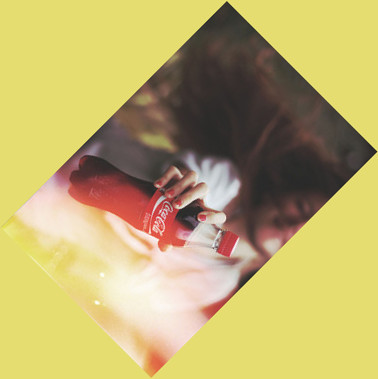## Shade¶

New in version 0.5.0.

Creates a 3D effect by simulating light from source where aziumth controls the X/Y angle, and elevation controls the Z angle. You can also determine of the resulting image should be transformed to grayscale by passing gray boolean.

from wand.image import Image

with Image(filename="hummingbird.jpg") as left:
with left.clone() as right:
right.shade(gray=True,
azimuth=286.0,
elevation=45.0)
left.extent(width=left.width*2)
left.composite(right, top=0, left=right.width)
left.save(filename="hummingbird-shade.jpg")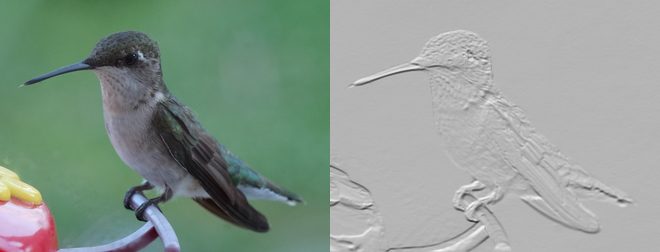## Sketch¶

New in version 0.5.3.

Simulates an artist sketch drawing. Also see Charcoal.

from wand.image import Image

with Image(filename="hummingbird.jpg") as left:
with left.clone() as right:
right.transform_colorspace("gray")
right.sketch(0.5, 0.0, 98.0)
left.extent(width=left.width*2)
left.composite(right, top=0, left=right.width)
left.save(filename="hummingbird-sketch.jpg")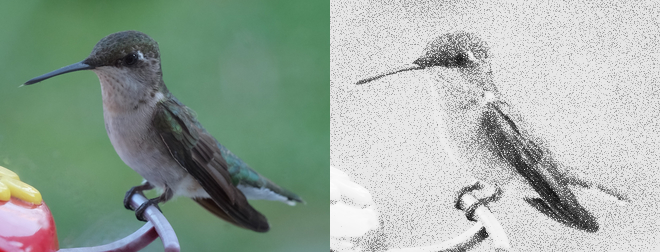## Spread¶

New in version 0.5.3.

Spread replaces each pixel with the a random pixel value found near by. The size of the area to search for a new pixel can be controlled by defining a radius.

from wand.image import Image

with Image(filename="hummingbird.jpg") as left:
with left.clone() as right:
right.spread(8.0)
left.extent(width=left.width*2)
left.composite(right, top=0, left=right.width)
left.save(filename="hummingbird-spread.jpg")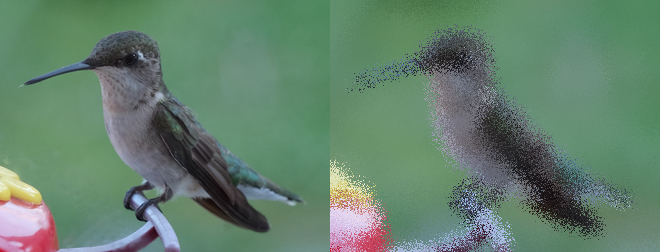## Statistic¶

New in version 0.5.3.

Similare to Spread, but replaces each pixel with the result of a mathematical operation performed against neighboring pixel values.

The type of statistic operation can be any of the following.

• 'gradient'
• 'maximum'
• 'mean'
• 'median'
• 'minimum'
• 'mode'
• 'nonpeak'
• 'root_mean_square'
• 'standard_deviation'

The size neighboring pixels to evaluate can be defined by passing width, and height kwargs.

from wand.image import Image

with Image(filename="hummingbird.jpg") as left:
with left.clone() as right:
right.statistic("median", width=8, height=5)
left.extent(width=left.width*2)
left.composite(right, top=0, left=right.width)
left.save(filename="hummingbird-statistic.jpg")